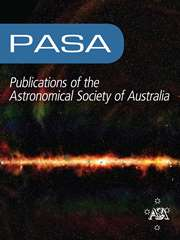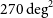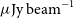Home
Hostname: page-component-684899dbb8-p6h7k Total loading time: 0.442 Render date: 2022-05-23T12:02:01.855Z Has data issue: true Feature Flags: { "shouldUseShareProductTool": true, "shouldUseHypothesis": true, "isUnsiloEnabled": true, "useRatesEcommerce": false, "useNewApi": true }Publications of the Astronomical Society of Australia

# The Evolutionary Map of the Universe pilot survey

Published online by Cambridge University Press:  07 September 2021

*
*Author for correspondence: Ray P. Norris, E-mail: raypnorris@gmail.com

## Abstract

We present the data and initial results from the first pilot survey of the Evolutionary Map of the Universe (EMU), observed at 944 MHz with the Australian Square Kilometre Array Pathfinder (ASKAP) telescope. The survey covers$270 \,\mathrm{deg}^2$ of an area covered by the Dark Energy Survey, reaching a depth of 25–30$\mu\mathrm{Jy\ beam}^{-1}$ rms at a spatial resolution of$\sim$ 11–18 arcsec, resulting in a catalogue of$\sim$ 220 000 sources, of which$\sim$ 180 000 are single-component sources. Here we present the catalogue of single-component sources, together with (where available) optical and infrared cross-identifications, classifications, and redshifts. This survey explores a new region of parameter space compared to previous surveys. Specifically, the EMU Pilot Survey has a high density of sources, and also a high sensitivity to low surface brightness emission. These properties result in the detection of types of sources that were rarely seen in or absent from previous surveys. We present some of these new results here.

## Keywords

Type
Research Article
© The Author(s), 2021. Published by Cambridge University Press on behalf of the Astronomical Society of Australia

## Access options

Get access to the full version of this content by using one of the access options below. (Log in options will check for institutional or personal access. Content may require purchase if you do not have access.)

## References

Abbott, T. M. C., et al. 2018, ApJS, 239, 18 CrossRefGoogle Scholar
Afonso, J., et al. 2011, ApJ, 743, 122 Google Scholar
Aguena, M., et al. 2021, MNRAS, 502, 4435 CrossRefGoogle Scholar
Bailer-Jones, C. A. L., Fouesneau, M., & Andrae, R. 2019, MNRAS, 490, 5615 CrossRefGoogle Scholar
Barnes, D. G., et al. 2001, MNRAS, 322, 486 Google Scholar
Becker, R. H., White, R. L., & Helfand, D. J. 1995, ApJ, 450, 559 CrossRefGoogle Scholar
Bera, A., Kanekar, N., Chengalur, J. N., & Bagla, J. S. 2019, ApJ, 882, L7 CrossRefGoogle Scholar
Bilicki, M., et al. 2016, ApJS, 225, 5 CrossRefGoogle Scholar
Boch, T., & Fernique, P. 2014, in Astronomical Data Analysis Software and Systems XXIII, ed. Manset, N., & Forshay, P., Astronomical Society of the Pacific Conference Series, vol. 485, 277Google Scholar
Bock, D. C. J., Large, M. I., & Sadler, E. M. 1999, AJ, 117, 1578 CrossRefGoogle Scholar
Boller, T., Freyberg, M. J., Trümper, J., Haberl, F., Voges, W., & Nandra, K. 2016, A&A, 588, A103 CrossRefGoogle Scholar
Bonaldi, A., Bonato, M., Galluzzi, V., Harrison, I., Massardi, M., Kay, S., De Zotti, G., & Brown, M. L. 2019, MNRAS, 482, 2 CrossRefGoogle Scholar
Bondi, M., Ciliegi, P., Schinnerer, E., Smolčić, V., Jahnke, K., Carilli, C., & Zamorani, G. 2008, ApJ, 681, 1129 CrossRefGoogle Scholar
Bonnarel, F., et al. 2000, A&AS, 143, 33 CrossRefGoogle Scholar
Brienza, M., et al. 2017, A&A, 606, A98 CrossRefGoogle Scholar
Briggs, D. S. 1995, PhD thesis, New Mexico Institute of Mining and Technology Socorro, New MexicoGoogle Scholar
Brown, M. J. I., Jannuzi, B. T., Floyd, D. J. E., & Mould, J. R. 2011, ApJ, 731, L41 CrossRefGoogle Scholar
Brüggen, M., et al. 2021, A&A, 647, A3 Google Scholar
Brunetti, G., & Jones, T. W. 2014, Int. J. Mod. Phys. D, 23, 1430007 CrossRefGoogle Scholar
Bulbul, E., et al. 2019, ApJ, 871, 50 CrossRefGoogle Scholar
Burenin, R. A., Vikhlinin, A., Hornstrup, A., Ebeling, H., Quintana, H., & Mescheryakov, A. 2007, ApJS, 172, 561 CrossRefGoogle Scholar
Calabretta, M. R., Staveley-Smith, L., Barnes, D. G., 2014, PASA, 31, e007 CrossRefGoogle Scholar
Chapman, J. M., Dempsey, J., Miller, D., Heywood, I., Pritchard, J., Sangster, E., Whiting, M., & Dart, M. 2017, in Astronomical Data Analysis Software and Systems XXV, ed. Lorente, N. P. F., Shortridge, K., & Wayth, R., Astronomical Society of the Pacific Conference Series, vol. 512, 73 Google Scholar
Condon, J. J. 1992, ARA&A, 30, 575 CrossRefGoogle Scholar
Condon, J. J., Cotton, W. D., Greisen, E. W., Yin, Q. F., Perley, R. A., Taylor, G. B., & Broderick, J. J. 1998, AJ, 115, 1693 CrossRefGoogle Scholar
Condon, J. J., Cotton, W. D., & Broderick, J. J. 2002, AJ, 124, 675 CrossRefGoogle Scholar
Cornwell, T. J., Golap, K., & Bhatnagar, S. 2008, IEEE J. Selected Top. Sig. Process., 2, 647 Google Scholar
Cotton, W. D., et al. 2020, MNRAS, 495, 1271 Google Scholar
Cutri, R. M., et al. 2021, VizieR Online Data Catalog, p. II/328Google Scholar
Dahlem, M., Ehle, M., Ryder, S. D., Vlajić, M., & Haynes, R. F. 2005, A&A, 432, 475 CrossRefGoogle Scholar
Davies, L. J. M., et al. 2017, MNRAS, 466, 2312 Google Scholar
Drlica-Wagner, A., et al. 2018, ApJS, 235, 33 Google Scholar
Duchesne, S. W., Johnston-Hollitt, M., Bartalucci, I., Hodgson, T., & Pratt, G. W. 2021, PASA, 38, e005 CrossRefGoogle Scholar
Eddington, A. S. 1913, MNRAS, 73, 359 CrossRefGoogle Scholar
Eddington, A. S. 1940, MNRAS, 100, 354 CrossRefGoogle Scholar
Ekers, R. D. 1970, AuJPh, 23, 217 CrossRefGoogle Scholar
Fanaroff, B. L., & Riley, J. M. 1974, MNRAS, 167, 31P CrossRefGoogle Scholar
Ferrand, G. 2019, Slicing the LCH space, Available at https://nbviewer.jupyter.org/github/gillesferrand/colourspace/blob/master/4.slices.ipynb (accessed 10 May 2021)Google Scholar
Ferrari, C., Govoni, F., Schindler, S., Bykov, A. M., & Rephaeli, Y. 2008, SSRv, 134, 93 CrossRefGoogle Scholar
Gaia Collaboration et al. 2016, A&A, 595, A2 Google Scholar
Gaia Collaboration et al. 2021, A&A, 649, A1 CrossRefGoogle Scholar
Giacintucci, S., Markevitch, M., Cassano, R., Venturi, T., Clarke, T. E., Kale, R., & Cuciti, V. 2019, ApJ, 880, 70 CrossRefGoogle Scholar
Gordon, Y. A., et al. 2021, arXiv e-prints, p. arXiv:2102.11753Google Scholar
Gregory, P. C., Vavasour, J. D., Scott, W. K., & Condon, J. J. 1994, ApJS, 90, 173 CrossRefGoogle Scholar
Hale, C. L., Jarvis, M. J., Delvecchio, I., Hatfield, P. W., Novak, M., Smolčić, V., & Zamorani, G. 2018, MNRAS, 474, 4133 CrossRefGoogle Scholar
Hay, S., O’Sullivan, J., Kot, J., & Granet, C. 2006, in The European Conference on Antennas and Propagation: EuCAP 2006, ed. Lacoste, H., & Ouwehand, L., Special Publication, ESA, vol. 626, 663Google Scholar
Healey, S. E., Romani, R. W., Taylor, G. B., Sadler, E. M., Ricci, R., Murphy, T., Ulvestad, J. S., & Winn, J. N. 2007, ApJS, 171, 61 CrossRefGoogle Scholar
Heesen, V., Brinks, E., Leroy, A. K., Heald, G., Braun, R., Bigiel, F., & Beck, R. 2014, AJ, 147, 103 CrossRefGoogle Scholar
Heesen, V., Dettmar, R.-J., Krause, M., Beck, R., & Stein, Y. 2016, MNRAS, 458, 332 CrossRefGoogle Scholar
Heesen, V., et al. 2018, MNRAS, 474, 5049 Google Scholar
Heywood, I., et al. 2016, MNRAS, 460, 4433 CrossRefGoogle Scholar
Hopkins, A. M., Afonso, J., Chan, B., Cram, L. E., Georgakakis, A., & Mobasher, B. 2003, AJ, 125, 465 CrossRefGoogle Scholar
Hotan, A. W., et al. 2021, PASA, 38, e009 Google Scholar
Huynh, M., Dempsey, J., Whiting, M. T., & Ophel, M. 2020, in Astronomical Data Analysis Software and Systems XXVII. ed. Ballester, P., Ibsen, J., Solar, M., Shortridge, K., Astronomical Society of the Pacific Conference Series, vol. 522, 263Google Scholar
HyeongHan, K., et al. 2020, ApJ, 900, 127 CrossRefGoogle Scholar
Ishwara-Chandra, C. H., & Saikia, D. J. 1999, MNRAS, 309, 100 CrossRefGoogle Scholar
Itten, J. 1970, The Elements of Colour. Van Nostrand Reinhold Company, Toronto Google Scholar
Jarrett, T. H., et al. 2017, ApJ, 836, 182 CrossRefGoogle Scholar
Johnston, S., et al. 2007, PASA, 24, 174 CrossRefGoogle Scholar
Johnston, S., et al. 2008, ExA, 22, 151 CrossRefGoogle Scholar
Jones, P. A., & McAdam, W. B. 1992, ApJS, 80, 137 CrossRefGoogle Scholar
Jones, D. H., et al. 2004, MNRAS, 355, 747 CrossRefGoogle Scholar
Jones, D. H., et al. 2009, MNRAS, 399, 683 Google Scholar
Koribalski, B. S., & López-Sánchez, á. R. 2009, MNRAS, 400, 1749 CrossRefGoogle Scholar
Koribalski, B. S., et al. 2004, AJ, 128, 16 Google Scholar
Koribalski, B. S., et al. 2020, Ap&SS, 365, 118 Google Scholar
Koribalski, B. S., Norris, R. P., Andernach, H., Rudnick, L., Shabala, S., Filipovic, M., & Lenc, E. 2021, MNRAS, 505, L11 CrossRefGoogle Scholar
Kużmicz, A., Jamrozy, M., Bronarska, K., Janda-Boczar, K., & Saikia, D. J. 2018, ApJS, 238, 9 CrossRefGoogle Scholar
Lacy, M., et al. 2020, PASP, 132, 035001 Google Scholar
Landy, S. D., & Szalay, A. S. 1993, ApJ, 412, 64 CrossRefGoogle Scholar
Leahy, D. A., et al. 2019, PASA, 36, e024 Google Scholar
Mahatma, V. H., et al. 2018, MNRAS, 475, 4557 CrossRefGoogle Scholar
Mancuso, C., et al. 2017, ApJ, 842, 95 CrossRefGoogle Scholar
Mandal, S., et al. 2021, A&A, 648, A5 Google Scholar
Marocco, F., et al. 2021, ApJS, 253, 8 CrossRefGoogle Scholar
Mauch, T., & Sadler, E. M. 2007, MNRAS, 375, 931 CrossRefGoogle Scholar
Mauch, T., Murphy, T., Buttery, H. J., Curran, J., Hunstead, R. W., Piestrzynski, B., Robertson, J. G., & Sadler, E. M. 2003, MNRAS, 342, 1117 CrossRefGoogle Scholar
McConnell, D., et al. 2016, PASA, 33, e042 Google Scholar
McConnell, D., et al. 2020, PASA, 37, e048 CrossRefGoogle Scholar
Meyer, M. J., et al. 2004, MNRAS, 350, 1195 CrossRefGoogle Scholar
Mocanu, L. M., et al. 2013, ApJ, 779, 61 CrossRefGoogle Scholar
Molnár, D. C., et al. 2021, MNRAS, 504, 118 CrossRefGoogle Scholar
Monroe, T. R., Prochaska, J. X., Tejos, N.,Worseck, G., Hennawi, J. F., Schmidt, T., Tumlinson, J., & Shen, Y. 2016, AJ, 152, 25 CrossRefGoogle Scholar
Murphy, E. J. 2009, ApJ, 706, 482 CrossRefGoogle Scholar
Murphy, T., et al. 2010, MNRAS, 402, 2403 Google Scholar
Murphy, E. J., et al. 2011, ApJ, 737, 67 CrossRefGoogle Scholar
Nawaz, M. A., Bicknell, G. V., Wagner, A. Y., Sutherland, R. S., & McNamara, B. R. 2016, MNRAS, 458, 802 CrossRefGoogle Scholar
Nordgren, T. E., Chengalur, J. N., Salpeter, E. E., & Terzian, Y. 1997, AJ, 114, 913 CrossRefGoogle Scholar
Norris, R. P. 2017a, NatAs, 1, 671 CrossRefGoogle Scholar
Norris, R. P. 2017b, PASA, 34, e007 Google Scholar
Norris, R. P., et al. 2006, AJ, 132, 2409 CrossRefGoogle Scholar
Norris, R. P., et al. 2011, PASA, 28, 215 CrossRefGoogle Scholar
Norris, R. P., et al. 2021, PASA, 38, e003 Google Scholar
O’Dea, C. P. 1998, PASP, 110, 493 CrossRefGoogle Scholar
O’Dea, C. P., & Saikia, D. J. 2021, A&A Rev., 29, 3 Google ScholarPubMed
Piffaretti, R., Arnaud, M., Pratt, G. W., Pointecouteau, E., & Melin, J. B. 2011, A&A, 534, A109 CrossRefGoogle Scholar
Pillepich, A., Reiprich, T. H., Porciani, C., Borm, K., & Merloni, A. 2018, MNRAS, 481, 613 CrossRefGoogle Scholar
Planck Collaboration et al. 2020, A&A, 641, A6 Google Scholar
Prandoni, I., Gregorini, L., Parma, P., de Ruiter, H. R., Vettolani, G., Wieringa, M. H., & Ekers, R. D. 2001, A&A, 365, 392 CrossRefGoogle Scholar
Prandoni, I., Parma, P., Wieringa, M. H., de Ruiter, H. R., Gregorini, L., Mignano, A., Vettolani, G., & Ekers, R. D. 2006, A&A, 457, 517 CrossRefGoogle Scholar
Prandoni, I., Guglielmino, G., Morganti, R., Vaccari, M., Maini, A., Röttgering, H. J. A., Jarvis, M. J., & Garrett, M. A. 2018, MNRAS, 481, 4548 CrossRefGoogle Scholar
Predehl, P., et al. 2021, A&A, 647, A1 Google Scholar
Quici, B., et al. 2021, PASA, 38, e008 Google Scholar
Rau, U., & Cornwell, T. J. 2011, A&A, 532, A71 CrossRefGoogle Scholar
Rau, U., Bhatnagar, S., Voronkov, M. A., & Cornwell, T. J. 2009, IEEE Proc., 97, 1472 CrossRefGoogle Scholar
Reiprich, T. H., et al. 2021, A&A, 647, A2 Google Scholar
Rudnick, L. 2002, PASP, 114, 427 CrossRefGoogle Scholar
Sabater, J., et al. 2019, A&A, 622, A17 Google Scholar
Saripalli, L., Subrahmanyan, R., Thorat, K., Ekers, R. D., Hunstead, R. W., Johnston, H. M., & Sadler, E. M. 2012, ApJS, 199, 27 CrossRefGoogle Scholar
Scheuer, P. A. G. 1957, Proce. Cambridge Philos. Soc., 53, 764 Google Scholar
Schilizzi, R. T., & McAdam, W. B. 1975, MmRAS, 79, 1 Google Scholar
Siewert, T. M., et al. 2020, A&A, 643, A100 Google Scholar
Tabatabaei, F. S., et al. 2017, ApJ, 836, 185 CrossRefGoogle Scholar
Taylor, M. B., 2005, in Astronomical Society of the Pacific Conference Series, vol. 347, Astronomical Data Analysis Software and Systems XIV, ed. Shopbell, P., Britton, M., & Ebert, R., 29Google Scholar
Turner, R. J., Rogers, J. G., Shabala, S. S., & Krause, M. G. H. 2018, MNRAS, 473, 4179 CrossRefGoogle Scholar
Urry, C. M., & Padovani, P. 1995, PASP, 107, 803 CrossRefGoogle Scholar
Vernstrom, T., et al. 2014, MNRAS, 440, 2791 CrossRefGoogle Scholar
White, R. L., Becker, R. H., Helfand, D. J., & Gregg, M. D. 1997, ApJ, 475, 479 CrossRefGoogle Scholar
White, S. V., et al. 2020a, PASA, 37, e017 Google Scholar
White, S. V., et al. 2020b, PASA, 37, e018 Google Scholar
Whiting, M. T. 2020, in Astronomical Data Analysis Software and Systems XXVII, ed. Ballester, P., Ibsen, J., Solar, M., & Shortridge, K., Astronomical Society of the Pacific Conference Series, vol. 522, 469Google Scholar
Whiting, M., & Humphreys, B. 2012, PASA, 29, 371 CrossRefGoogle Scholar
Whiting, M., Voronkov, M., Mitchell, D., & Askap Team, 2017, Early Science Pipelines for ASKAP, 431Google Scholar
Whittam, I. H., et al. 2013, MNRAS, 429, 2080 CrossRefGoogle Scholar
Wilman, R. J., et al. 2008, MNRAS, 388, 1335 Google Scholar
Wong, O. I., Meurer, G. R., Zheng, Z., Heckman, T. M., Thilker, D. A., & Zwaan, M. A. 2016, MNRAS, 460, 1106 CrossRefGoogle Scholar
Zhou, R., et al. 2021, MNRAS, 501, 3309 CrossRefGoogle Scholar
Zinn, P. C., Middelberg, E., Norris, R. P., Hales, C. A., Mao, M. Y., & Randall, K. E. 2012, A&A, 544, A38 CrossRefGoogle Scholar
Zou, H., Gao, J., Zhou, X., & Kong, X. 2019, ApJS, 242, 8 CrossRefGoogle Scholar
Zou, H., Gao, J., Zhou, X., & Kong, X. 2020, VizieR Online Data Catalog, J/ApJS/242/8CrossRefGoogle Scholar
van Weeren, R. J., de Gasperin, F., Akamatsu, H., Brüggen, M., Feretti, L., Kang, H., Stroe, A., & Zandanel, F. 2019, SSRv, 215, 16 CrossRefGoogle Scholar
5
Cited by

# Save article to Kindle

Note you can select to save to either the @free.kindle.com or @kindle.com variations. ‘@free.kindle.com’ emails are free but can only be saved to your device when it is connected to wi-fi. ‘@kindle.com’ emails can be delivered even when you are not connected to wi-fi, but note that service fees apply.

Find out more about the Kindle Personal Document Service.

The Evolutionary Map of the Universe pilot survey
Available formats
×

# Save article to Dropbox

To save this article to your Dropbox account, please select one or more formats and confirm that you agree to abide by our usage policies. If this is the first time you used this feature, you will be asked to authorise Cambridge Core to connect with your Dropbox account. Find out more about saving content to Dropbox.

The Evolutionary Map of the Universe pilot survey
Available formats
×

# Save article to Google Drive

To save this article to your Google Drive account, please select one or more formats and confirm that you agree to abide by our usage policies. If this is the first time you used this feature, you will be asked to authorise Cambridge Core to connect with your Google Drive account. Find out more about saving content to Google Drive.

The Evolutionary Map of the Universe pilot survey
Available formats
×
×